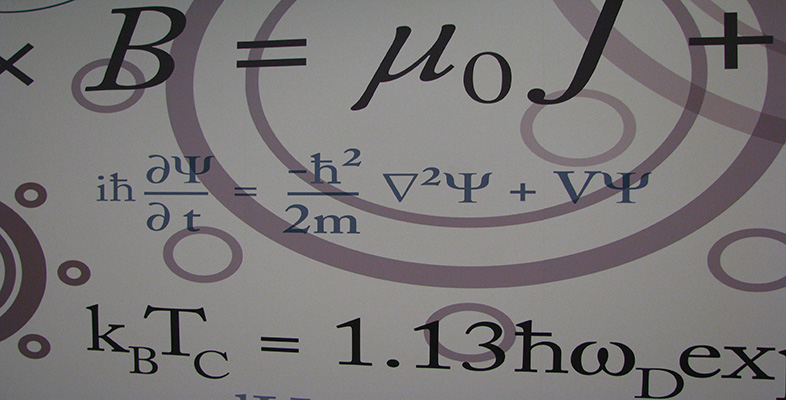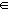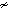Number systems

This free course is available to start right now. Review the full course description and key learning outcomes and create an account and enrol if you want a free statement of participation.

Free course

Relations

We shall use the symbol(known as tilde or twiddle) to represent a relation between two elements of a set.

Some texts use ρ, rather than, for an arbitrary relation. Certain relations have special symbols; for example,

• < means is less than,

• = means is equal to.

Definition

We say thatis a relation on a set X if, whenever x, yX, the statement xy is either true or false.

• If xy is true, then x is related to y.

• If xy is false, then x is not related to y and we write xy.

The statement xy can be read as ‘x is related to y’ or ‘x twiddles y’.

M208_6